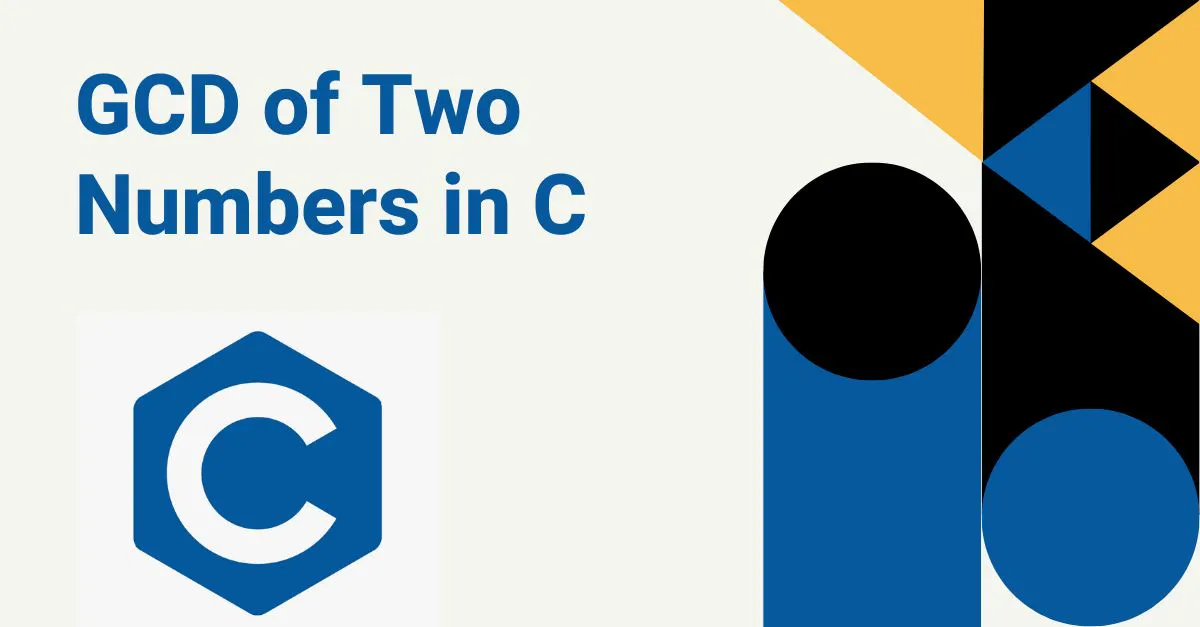How to Get the GCD Of Two Numbers in Cby@ankitdixit

# How to Get the GCD Of Two Numbers in C

July 8th 2022
6 min🖨️

The greatest common common divisor (GCD) is the largest positive integer that is also a common diviisor of a given set of positive integers. It is also known as the highest common factor (HCF) or the greatest common factor. The GCD of any two numbers is never negative or zero since the smallest positive. integer shared by any two. numbers is always 1. We can reduce the time complexity of this algorithm by using an efficient approach to find GCD. The Euclidean Algorithm is based on the above algorithm.### @ankitdixit

Ankit Dixit